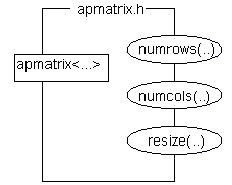The apmatrix class

The apmatrix class implements resizable two-dimensional arrays with safe subscripting. It is similar to the apvector class with the following differences --

• the constructors and the `resize(..)` function take two arguments, rows and cols, instead of one, size
• the `length( )` function is replaced by two functions, `numrows( )` and `numcols( )`.Here are examples of the four apmatrix constructors - ``` apmatrix words; // empty (zero-size) 2-D array of apstrings apmatrix table(10,2); // matrix of doubles with 10 rows and 2 columns apmatrix board(8,8,'X'); // 8 by 8 matrix of chars filled with 'X' ``````apmatrix newboard ( board); // second 8 by 8 matrix copied from board x. ```

Here are some more examples of how to use apmatrices in a program -

```#include "apmatrix.h"		// include the header file
int rows = table.numrows( );	// determine the current number of rows of the matrix
int cols = table.numcols( );	// determine the current number of columns of the matrix
table.resize(5,3);		// set new dimensions of the matrix, truncating extra
// elements and filling new elements with default values,
// (zeros for int, double, char types)
table = 4.2;		// store 4.2 in the first row, second column of the matrix
// at runtime checks that subscripts are within legal range
```
These two prototype functions show how to always pass matrix objects by reference.
```void fillMatrix(apmatrix &table);
// Note that the & must be explicitly used when a matrix object is passed by reference.

void displayMatrix(const apmatrix &table);
// To avoid unnecessary copying, do not pass an object by value .
// Pass by reference using the keyword const to ensure the object cannot be changed
```

Continue to:  Unit 4  / Prev  / Next